# Blog

Statistics for Sustainable Development > Blog > Warming Stripes: A problem solving task in R

Technical Pieces

## Introduction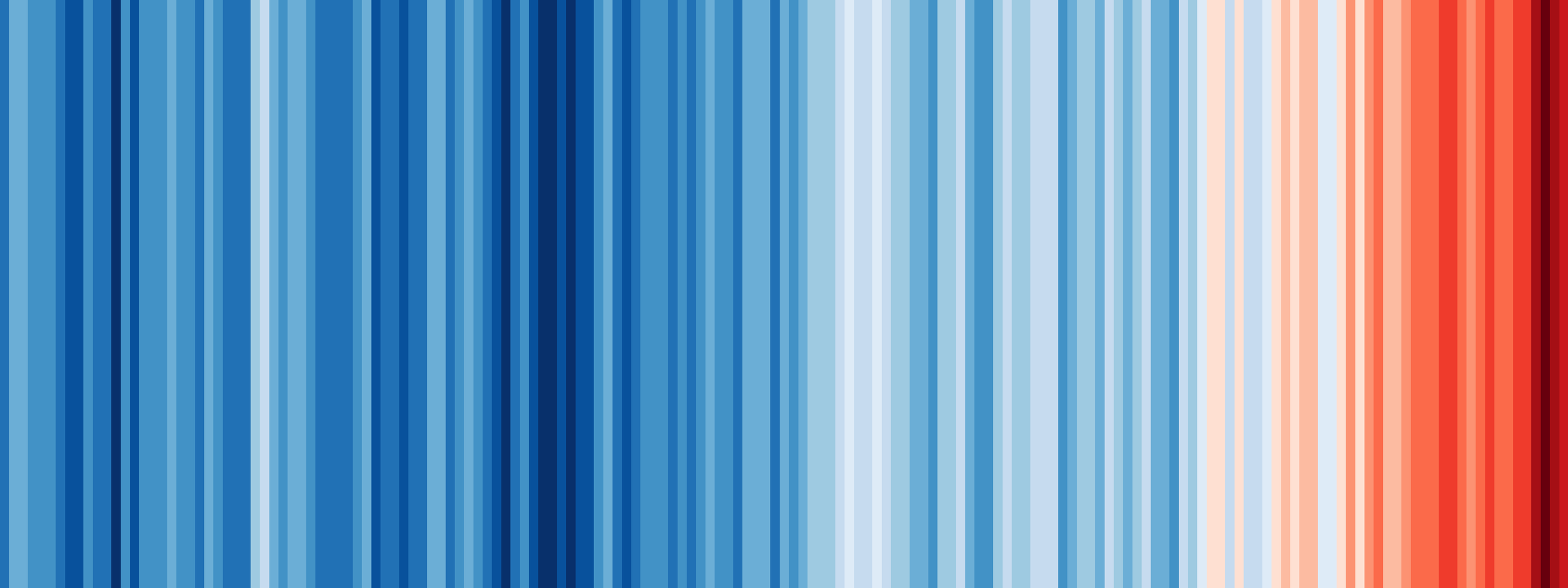One of the most famous and powerful pieces of data visualisation in recent years, originated just a few hundred metres away from the Stats4SD offices - at the University of Reading. In this blog I am going to show you how, using only some fairly standard tidyverse R functions, you can recreate this plot for any of your own data- and indeed start customising it further should you so choose!

If you would rather watch me talk instead of reading this blog - you can watch a video of me talking through this solution here:

The ‘Warming Stripes’ graph, created by Professor Ed Hawkins, sparked huge amounts of interest, and debate, thanks to its innovative and striking yet easily understood presentation. You can’t go far on the University of Reading campus without seeing it plastered on a poster, sign or even a face-mask.

It’s a way of displaying trends in annual average temperatures over time for a particular location. Depending on the location, and the time range of the data, it is extremely common to see a plot like the one above - highlighting clearly how average temperatures have increased.

It is possible to make these plots for ourselves in R, with almost identical formatting to the famous style, using only some data importation steps, and some commonly used functions from `dplyr` & `ggplot2`. But it will require some careful thinking and problem solving to get to that point! I am assuming if you are reading this blog you have come across `dplyr` and `ggplot2` before. If not - don’t worry so much about following the exact code - the key idea here is to think of this as a problem solving exercise using R. We do not ever find the solution to a complex problem fully formed - instead we need to break it down into multiple steps, and then build these up in a sensible sequence.

Once we start to get comfortable thinking of R as a programming language, rather than just a data analysis software, then our options for producing customised analyses and visualisations becomes almost limitless. This is one of the reasons why I love R, and particularly the tidyverse functions, as it encourages you to experiment and try things out with the data. Not every idea turns out as successfully as the warming stripes plots, but without the ability to experiment in this way we would never be able to create something of that sort.

If you want to follow through with the example beyond this point, then it’s probably best if you have already used R, and are somewhat familiar with tidyverse functions. If not, hopefully my introduction has convinced you to start learning! You can find some of our “Introduction to R and RStudio” videos here: https://www.youtube.com/playlist?list=PLK5PktXR1tmM_ISpxwoiXD2SioG3MWNqg

## Data & Methodology

We are going to use data from a weather station in Oxford, one of the longest running weather stations for which the data is easily available from the UK Met Service.

In the warming stripes plot we see one ‘stripe’ representing one year of data. The colour represents the difference between the yearly average temperature and the average temperature in the reference period, 1971-2000. This is known as the temperature anomaly. With our data we would want to make sure we only include those years where we have complete data available. If we are missing data in January, for example, this is going to give us biased estimates as we are missing some of the colder values.

Within the plot, increasingly dark shades of red represent values with an increasingly positive temperature anomaly; increasingly dark shades of blue represent an increasingly negative temperature anomaly. An anomaly of 0, or close to 0, should be shaded in white, so that the colour scale is symmetrical.

Before we try to write any code here, we should make sure we are confident with what we are trying to achieve visually as well. It’s worth taking a look at the examples from https://showyourstripes.info/ to give ourselves a target for how we will adjust the appearance of the plot.

We will need to use `dplyr` and `ggplot2`. So let’s make sure we remember to load them in to our session!

``````library(dplyr)
library(ggplot2)``````

## Stage 1: Import Data

You can find the version of the data I am using here: https://raw.githubusercontent.com/stats4sd/r2020_08Stripe/main/oxforddata_tidied.csv

(Note: There were a few steps needed to clean this data up from the raw version made publically available by the Met Office. You can watch a video of me talking through these steps here: https://youtu.be/6xG5U35pBFo )

With the tidied csv file we can import the data in a fairly standard way, using `read.csv`.

``oxford_data<-read.csv("oxforddata_tidied.csv")``

When we check the data we should make a note that there is a single missing value in one the columns we are going to need to use - the minimum temperature.

``summary(oxford_data)``
``````##       yyyy            mm             tmax            tmin
##  Min.   :1853   Min.   : 1.00   Min.   :-0.20   Min.   :-5.800
##  1st Qu.:1894   1st Qu.: 3.75   1st Qu.: 9.00   1st Qu.: 2.800
##  Median :1936   Median : 6.50   Median :13.80   Median : 5.600
##  Mean   :1936   Mean   : 6.50   Mean   :13.94   Mean   : 6.216
##  3rd Qu.:1978   3rd Qu.: 9.25   3rd Qu.:19.00   3rd Qu.:10.200
##  Max.   :2019   Max.   :12.00   Max.   :27.40   Max.   :15.700
##                                                 NA's   :1
##        af              rain             sun
##  Min.   : 0.000   Min.   :  0.50   Min.   : 18.2
##  1st Qu.: 0.000   1st Qu.: 31.48   1st Qu.: 72.3
##  Median : 1.000   Median : 49.75   Median :123.0
##  Mean   : 3.726   Mean   : 54.73   Mean   :128.2
##  3rd Qu.: 6.000   3rd Qu.: 74.80   3rd Qu.:173.6
##  Max.   :28.000   Max.   :192.90   Max.   :303.7
##  NA's   :1                         NA's   :912``````

## Stage 2: Manipulate Data

Before going any further, we should consider the structure of our data as it is now, and the structure of the data we need to be able to make a plot.

Right now the data we have is:

• One row per month
• Two columns for temperature (tmax and tmin)

The data we need for the plot would have:

• One row per year
• One column for temperature anomaly

So there are going to be quite a few steps needed here to get from A to B. We definitely want to try to work these out in advance before writing the code. Some people would find it useful to draw a diagram, to help think about what the steps are and what order they should be in.

There are broadly 5 steps needed, in the order that made the most sense to me:

2.1: Calculate a single monthly average temperature value

2.2: Calculate the average temperature from within the reference period

2.3: Group by year, and then obtaining annual averages of the average temperatures

2.4: Calculate the temperature anomaly for each year

2.5: Filter to a sensible range of years with complete data

### 2.1 Monthly average

To get the monthly average temperature we will need to combine the maximum `tmax` and minimum `tmin` columns in some way. The simplest option is to just take the average of these values.

This is a little trickier than it seems, since we need to take an average of values from multiple columns, unlike most of the time in R when we are taking the average of multiple rows.

The easiest way is to do this is simply go back to first principles and remember the formula for calculating a mean: add all the values, then divide the sum by the number of values. I can do this using `mutate()` to create a new column called `tmean`.

``````oxford_data<-
oxford_data %>%
mutate(tmean=(tmin+tmax)/2)``````

I am also going to over-write the existing object at this point by assigning the output to be an object called `oxford_data`. Since step 2.2 will not be inside a continuous pipe (although in the video solution, I did use a slightly more complicated method to do everything within a single pipe. The code for that alternative is included at the end of this solution.)

### 2.2: Reference period average

From the updated data created in 2.1, I will filter to just the years 1971-2000 and then get the mean value.

``````refmean=oxford_data %>%
filter(yyyy>=1971 & yyyy<=2000) %>%
summarise(mean=mean(tmean))``````

### 2.3: Yearly average

Using `group_by` and `summarise`, I can now calculate mean values for each year. Remember there was one missing value in our dataset. Because of the seasonality of temperature, if we use `na.rm=T` when calculating the mean temperature for the year, we will definitely have a biased estimate of the mean. Therefore it is probably safer to not use this option for calculating the mean, and leaving the mean for this year as an NA.

``````oxford_data %>%
group_by(yyyy) %>%
summarise(tmean=mean(tmean))``````
``````## # A tibble: 167 x 2
##     yyyy tmean
##    <int> <dbl>
##  1  1853  8.97
##  2  1854  9.69
##  3  1855  8.83
##  4  1856  9.53
##  5  1857 10.3
##  6  1858  9.50
##  7  1859 10.1
##  8  1860 NA
##  9  1861  9.76
## 10  1862  9.90
## # ... with 157 more rows``````

### 2.4: Temperature anomaly

Finally, we can calculate the temperature anomaly by subtracting the yearly averages from the reference period average:

``````oxford_data %>%
group_by(yyyy) %>%
summarise(tmean=mean(tmean)) %>%
mutate(anomaly=tmean-refmean\$mean)``````
``````## # A tibble: 167 x 3
##     yyyy tmean anomaly
##    <int> <dbl>   <dbl>
##  1  1853  8.97  -1.42
##  2  1854  9.69  -0.699
##  3  1855  8.83  -1.56
##  4  1856  9.53  -0.853
##  5  1857 10.3   -0.116
##  6  1858  9.50  -0.891
##  7  1859 10.1   -0.291
##  8  1860 NA     NA
##  9  1861  9.76  -0.628
## 10  1862  9.90  -0.482
## # ... with 157 more rows``````

Note that, even though there is only one value in the data frame `refmean`, we still need to refer to the column name to access this value.

### 2.5 Filter data

Because of the missing value in 1860, then it probably makes sense to only plot the years we have complete data for. So let’s restrict the plot to only 1861 and onwards.

``````oxford_plot_data<-
oxford_data %>%
group_by(yyyy) %>%
summarise(tmean=mean(tmean)) %>%
mutate(anomaly=tmean-refmean\$mean)%>%
filter(yyyy>=1861)``````

I also decided to assign this into a new object called `oxford_plot_data` at this point. I will then use this data for my plots!

## Stage 3: Plotting

### Make the initial plot

Let’s deconstruct the plot structure to understand more about how it works.

• We have year on the x axis
• A coloured ‘stripe’ for each year, (a bar of constant height)
• The colour represents the anomaly

So within `ggplot2` our solution will be

• Map `yyyy` to the x axis
• Map `anomaly` to the fill axis
• The variable going onto the y axis should not be a variable at all! We want a constant, so we can just set this to be an arbitrary value. We do need to set this to be equal to some value, so R knows how high to make each bar.
• Use `geom_col` not `geom_bar`, because we are setting the heights of the bars, rather than using bars to summarise counts, or other statistics, from data

This gives us:

``````ggplot(data=oxford_plot_data,aes(x=yyyy,y=1,fill=anomaly))+
geom_col()``````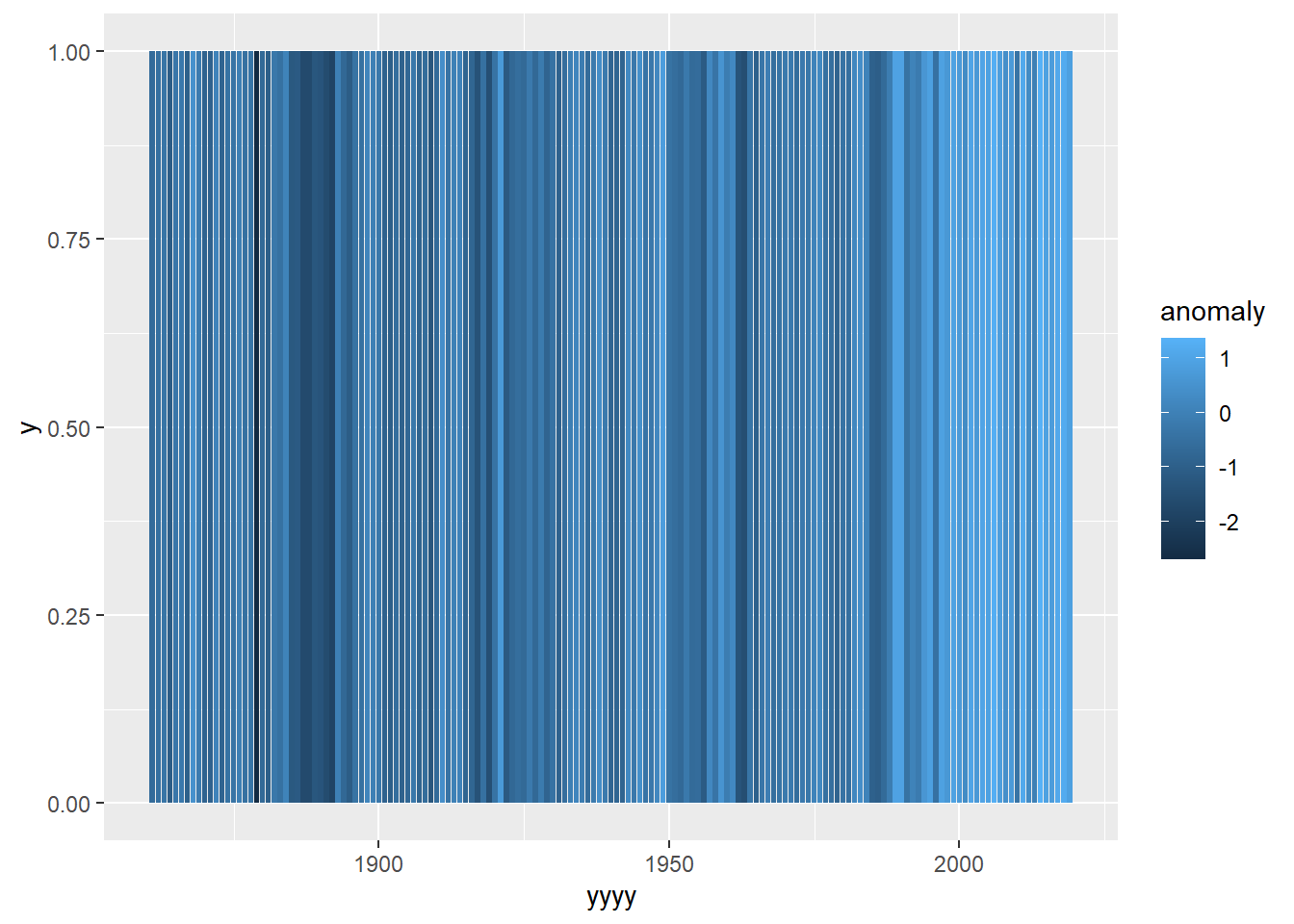Now we need to think about how to format this into the classic ‘warming bars’ style. There are a few things we need to do:

1. Change the colour palette so that low values are blue and high values are red and zero is white
2. Make the colour scale ‘symmetrical’. You can see from the legend that the zero value is not in the middle of scale.
3. Remove the small spaces between bars
4. Remove all the gridlines, axis labels, and backgrounds

### 3.1 Colour Palette

This is where we will need to use a `scale_` function, to modify the way the colours are allocated.

There are a few different scale functions for creating colour gradients depending on whether you need a single colour gradient, a double colour gradient or a n-level colour gradient. In this particular case we need a “double” gradient - because we want to set negative values to go from dark blue up to white (at zero).

Then from zero we want an additional gradient to go from white to dark red as the numbers become more positive. So the function we need is `scale_fill_gradient2`. In this we need to set the colour values for our “low”, “mid” and “high” extremes of colour.

``````ggplot(data=oxford_plot_data,aes(x=yyyy,y=1,fill=anomaly))+
geom_col()+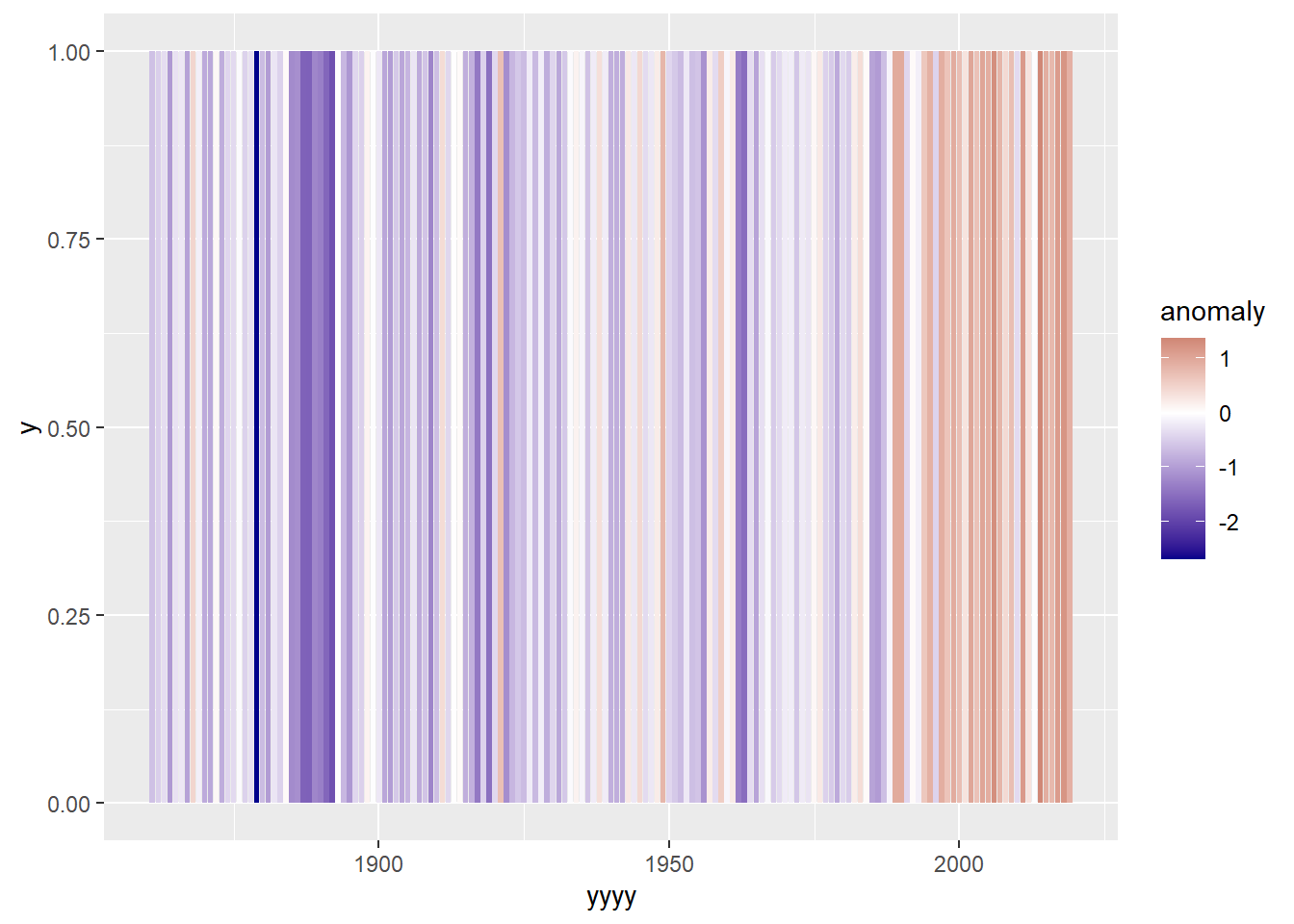You can see if you do not add in the “white” mid point, and just use the single red-blue gradient, using `scale_fill_gradient()` then the plot does not look quite right:

``````ggplot(data=oxford_plot_data,aes(x=yyyy,y=1,fill=anomaly))+
geom_col()+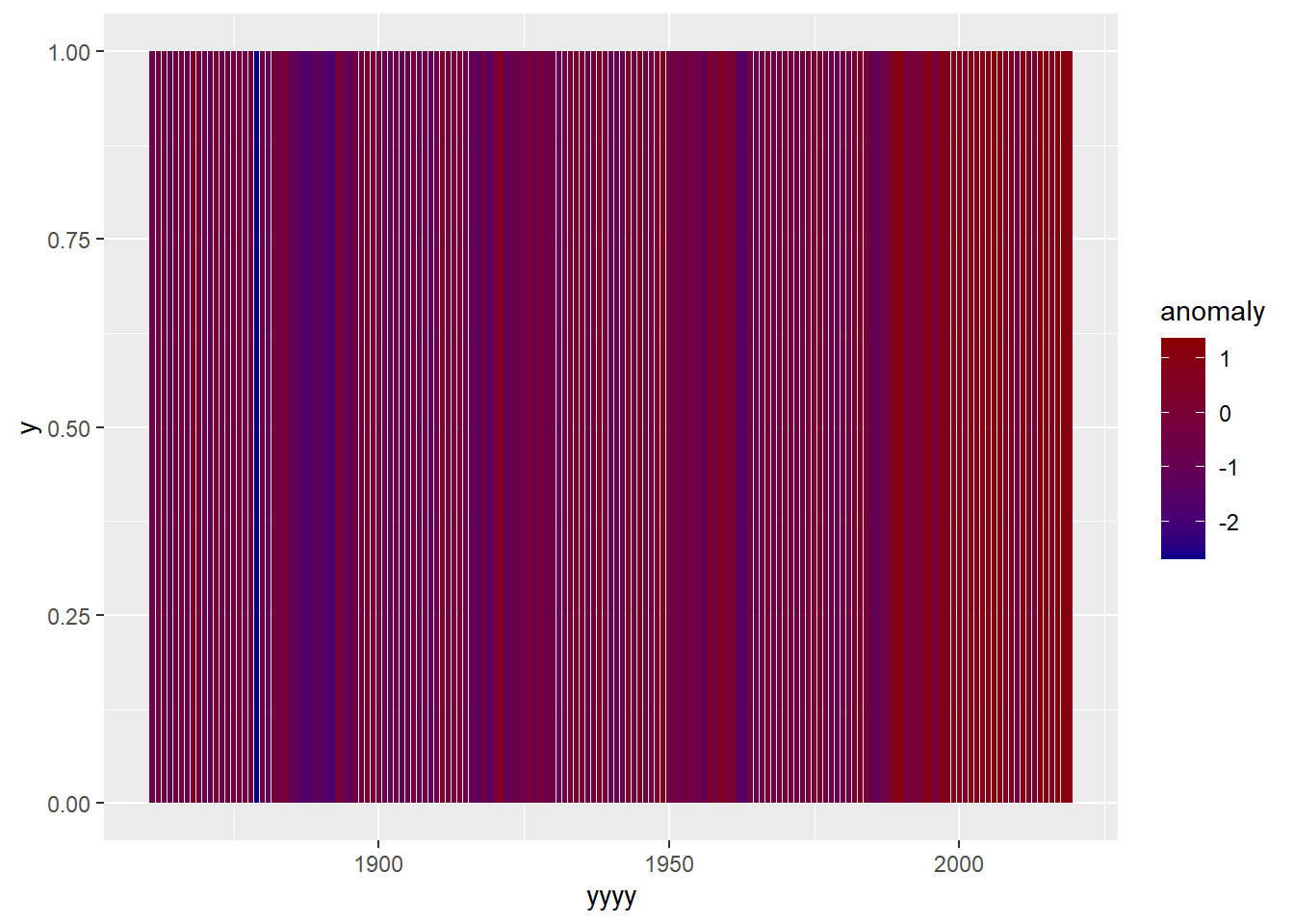An even better option here would be to use the new function `scale_fill_fermenter()`which works in a similar way to `scale_fill_brewer()` except with continuous variables being used to set colours instead. This is nice because the “RdBu” colour palette from colour brewer is exactly the colour palette used in the warming stripes plots. And this colour palette does already centre around a shade of white.

``````ggplot(data=oxford_plot_data,aes(x=yyyy,y=1,fill=anomaly))+
geom_col()+
scale_fill_fermenter(palette="RdBu")``````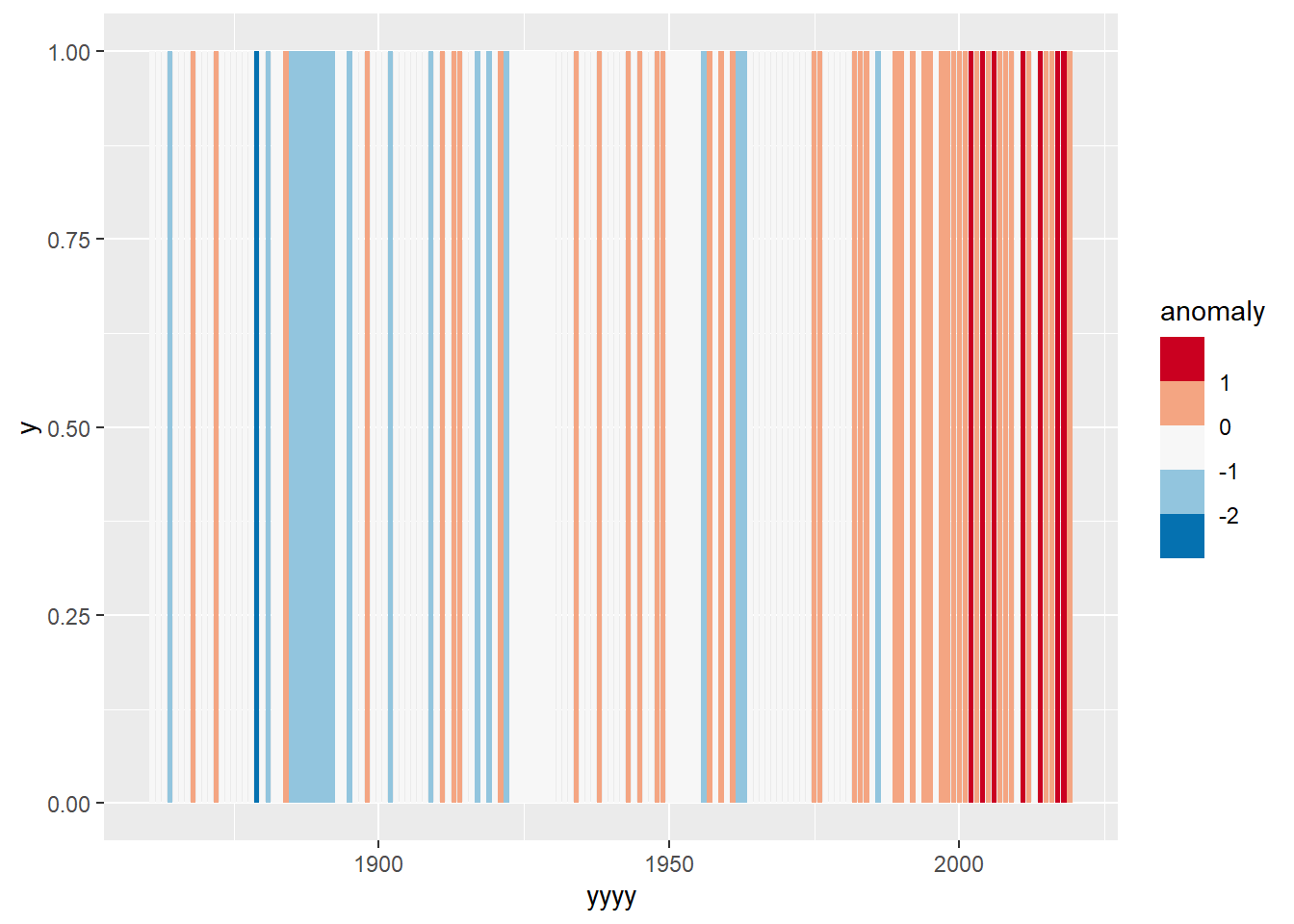### 3.2 Symmetrical colour scale

A little surprisingly, because of the default options within `scale_fill_gradient2`, we now have a symmetrical colour palette! The function defaults to a midpoint of zero, and stretches the colour assignments so that they are symmetrical. You can’t tell this from just looking at the plot, but the top level of ‘reds’ being plotted is not at the full extent of ‘darkred’, unlike the blue which does extend as far as possible. If we did want to make sure of this explicitly we could set the limits within the scale call, exactly as we might modify the limits of an x or y axis as we have seen previously.

``````ggplot(data=oxford_plot_data,aes(x=yyyy,y=1,fill=anomaly))+
geom_col()+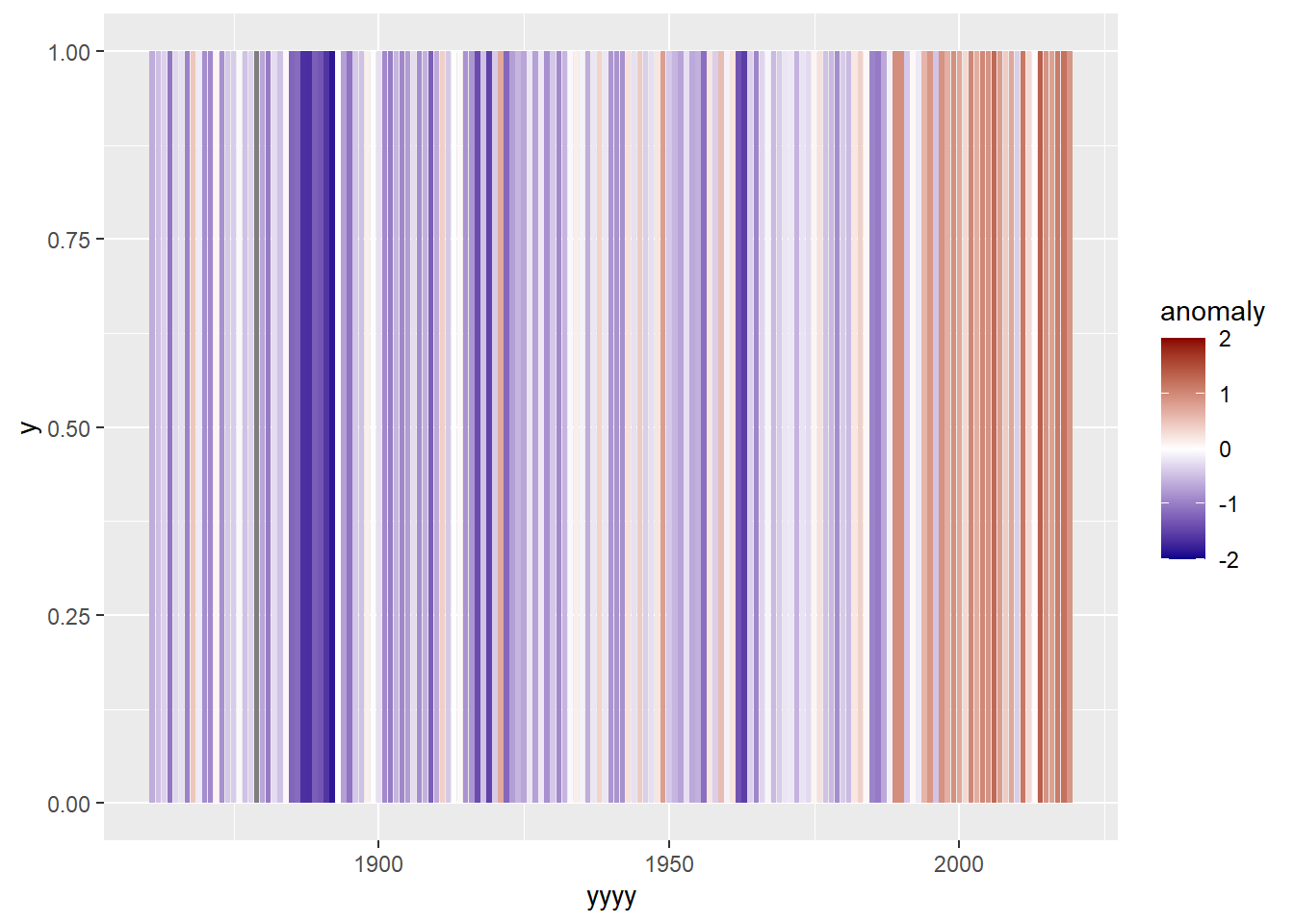The plot is identical, but you can see the darkest red in the legend is now a bit darker!

Within `scale_fill_fermenter` this is not automatic - so we would need to explicitly set limits, and make sure they are symmetrical.

``````ggplot(data=oxford_plot_data,aes(x=yyyy,y=1,fill=anomaly))+
geom_col()+
scale_fill_fermenter(palette="RdBu",limits=c(-1.5,1.5))``````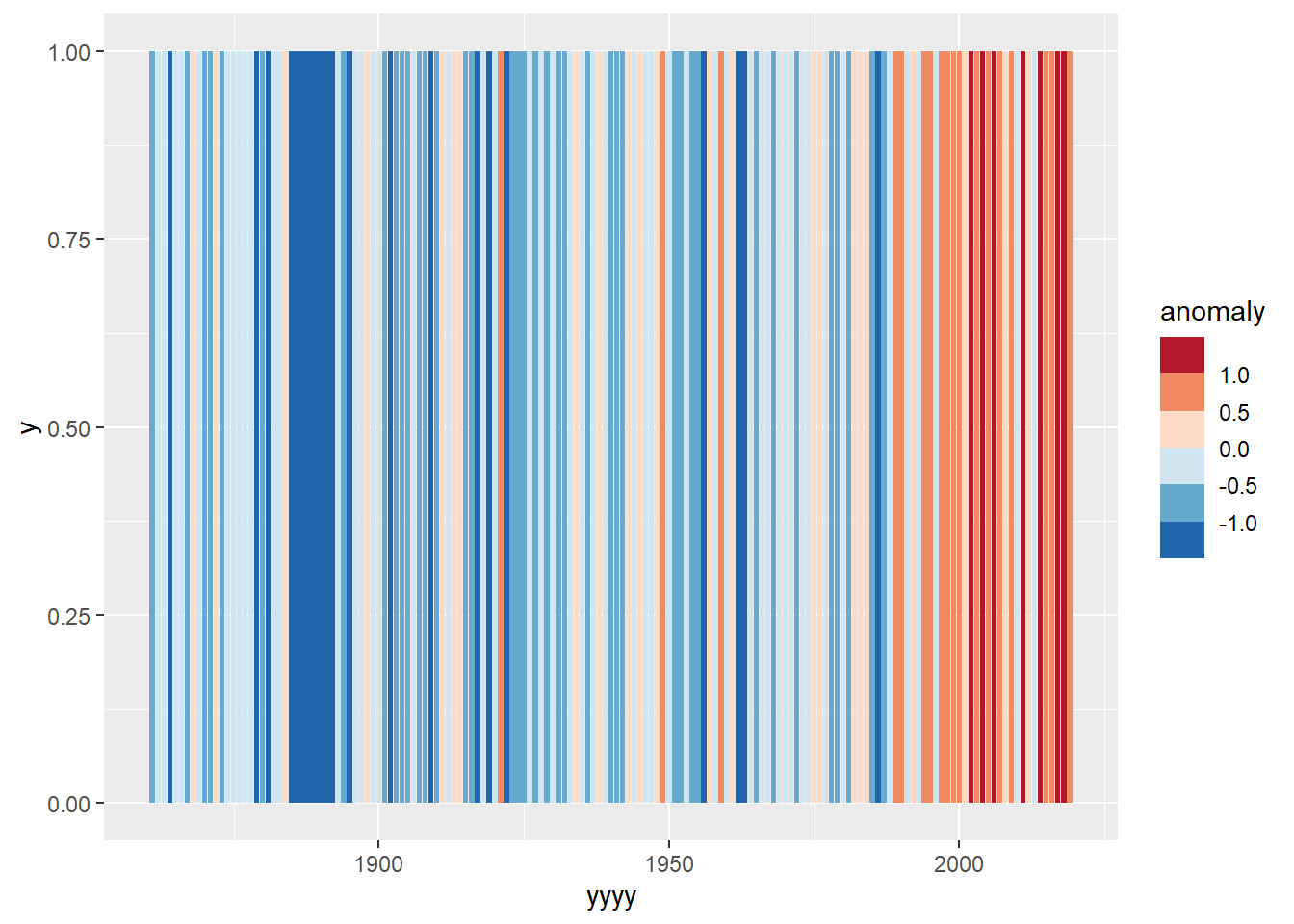### 3.3 Removing spaces

It may not be obvious without zooming in, but there are small spaces between each bar. This is because by default R sets bars to fill 90% of the width available, and leaves 5% spacing between bars. However no such spaces exist in the ‘classic’ warming stripes. Thankfully this is easy to resolve by adding the option width=1 into geom_col.

``````ggplot(data=oxford_plot_data,aes(x=yyyy,y=1,fill=anomaly))+
geom_col(width=1)+
scale_fill_fermenter(palette="RdBu",limits=c(-1.5,1.5))``````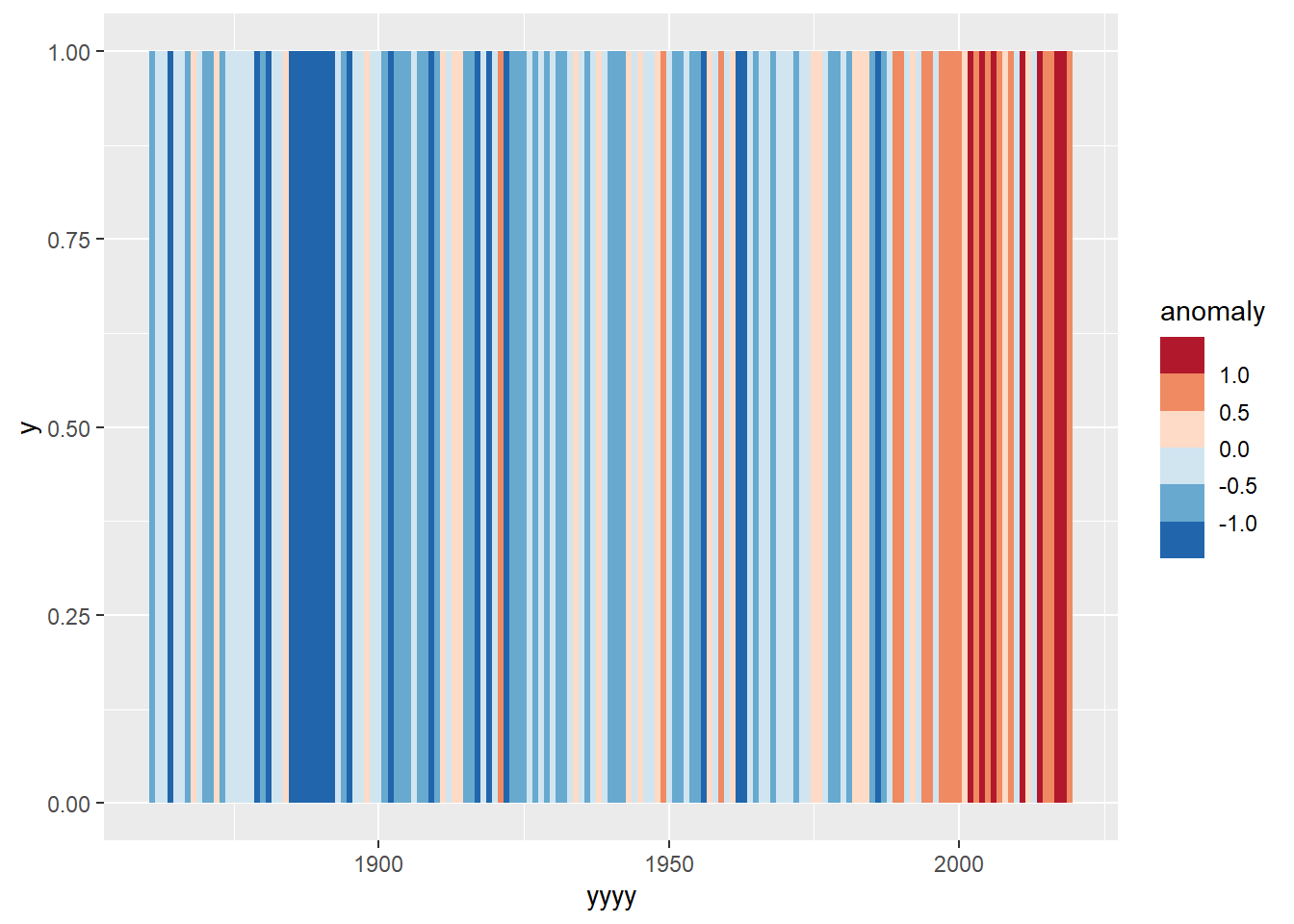### 3.4 Removing labels, gridlines etc.

With the `theme()` function we can manually change individual components. We could use this to individually remove the labels, and gridlines. But there is also a built in theme which will do this for us - `theme_void()`.

``````ggplot(data=oxford_plot_data,aes(x=yyyy,y=1,fill=anomaly))+
geom_col(width=1)+
scale_fill_fermenter(palette="RdBu",limits=c(-1.5,1.5))+
theme_void()``````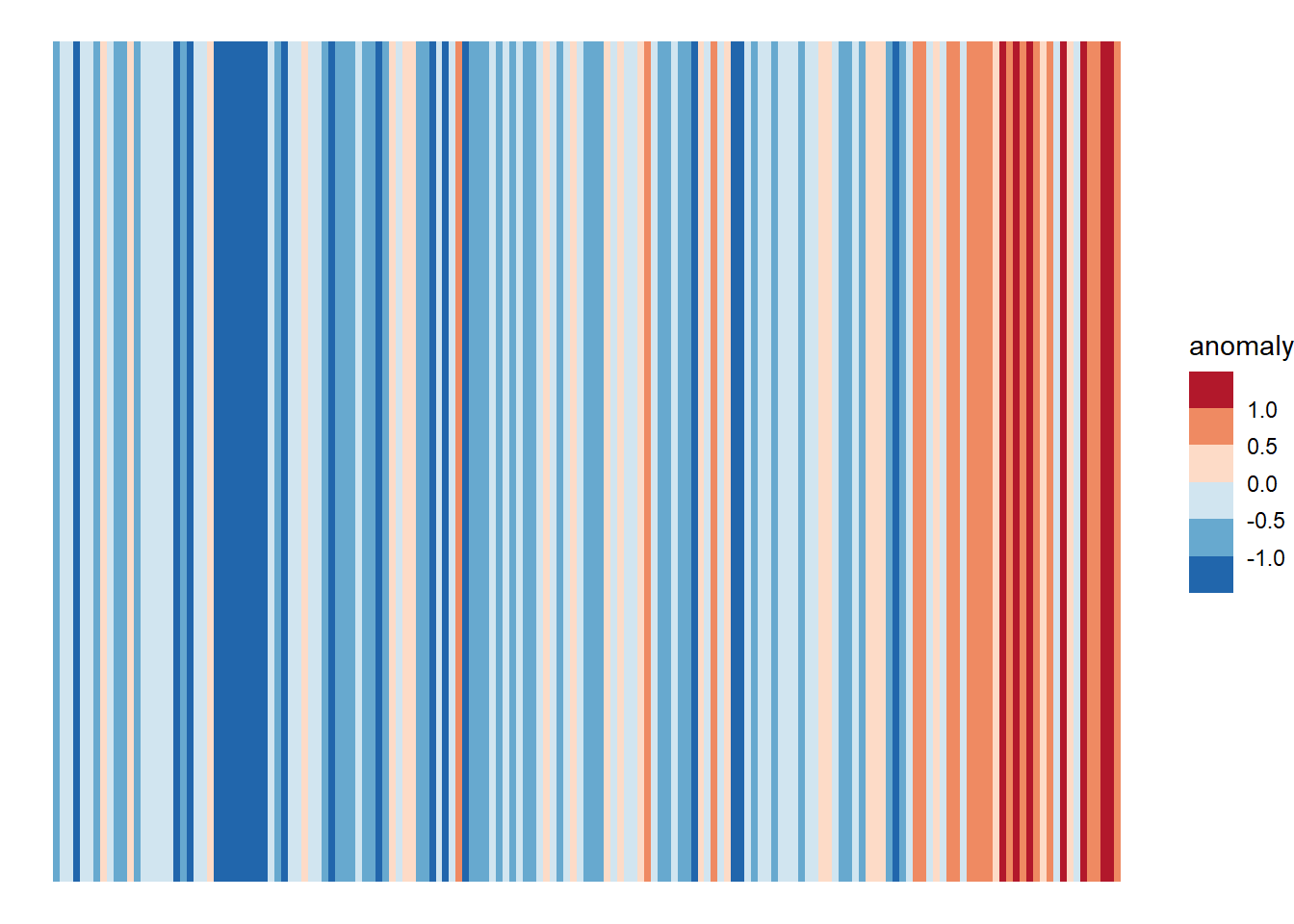We might also want to get rid of the legend, and add a title. Just to add a finishing touch

``````ggplot(data=oxford_plot_data,aes(x=yyyy,y=1,fill=anomaly))+
geom_col(width=1,show.legend = FALSE)+
scale_fill_fermenter(palette="RdBu",limits=c(-1.5,1.5))+
theme_void()+
labs(title="Oxford Warming Stripes",subtitle = "1861-2019")``````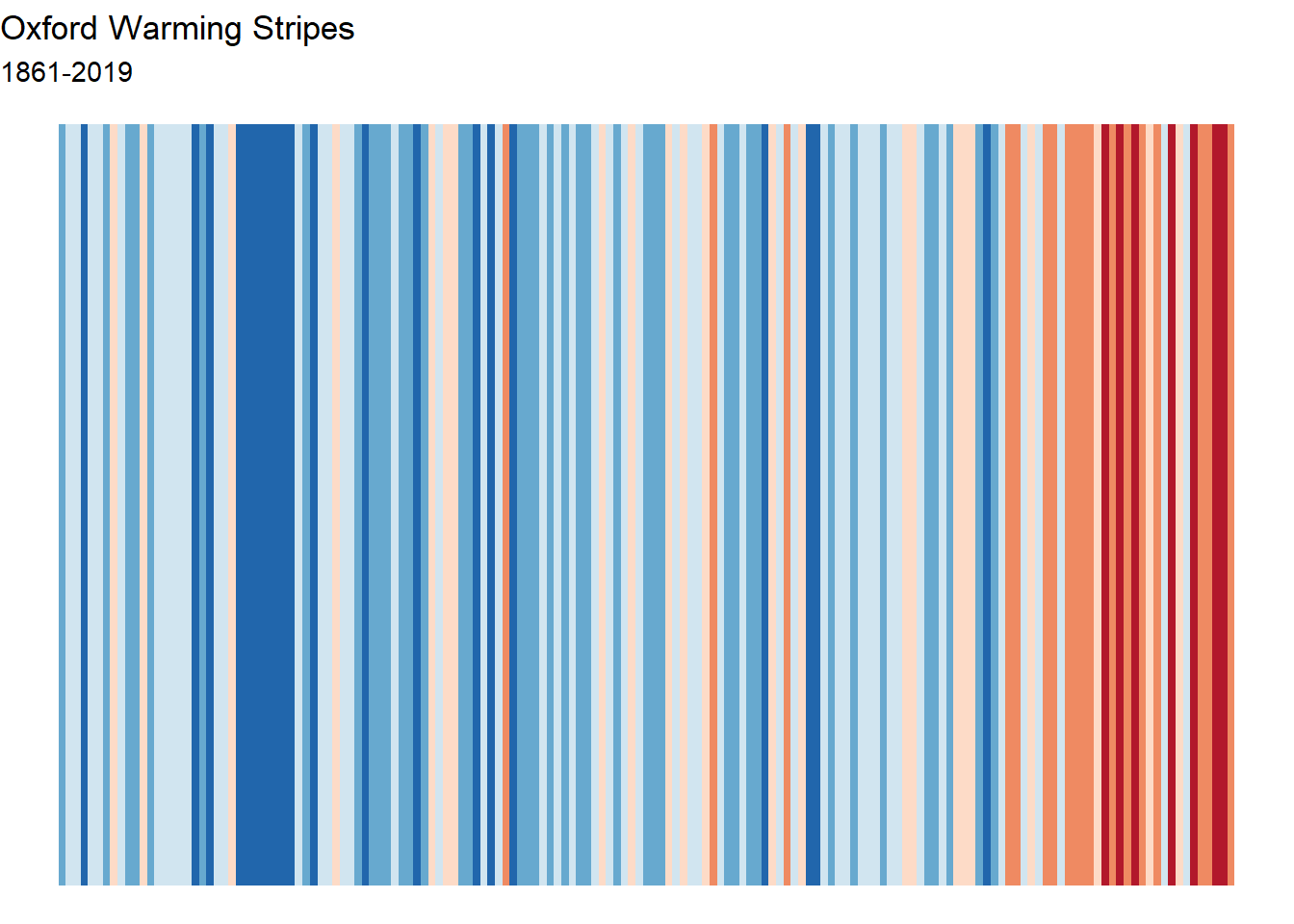Job done! Although I am sure there is plenty more we could keep tweaking around here!

## Conclusion

The key thing for me in this example is the power of linking together your data, with some flexible tools and being able to apply a little bit of creativity and problem solving. In order to really maximise the potential of any data analysis, all three things are needed. There is never one single ‘best’ way to analyse or present your data, and many data analysis software can - providing you build your own skills and are willing to challenge yourself to try to produce solutions that do not come straight ‘out of the box’.

## Appendix 1: Solution in one pipe

For the very adventurous! I don’t take exactly the same route in the video as I work through above. In the video I am demonstrating a method for doing the whole thing in one piped sequence. Which means there is one step which is a little more complex in the video, than the route outlined here. This is the same route used in the video solution.

``````oxford_data %>%
mutate(tave=(tmax+tmin)/2) %>%
group_by(yyyy) %>%
summarise(tave=mean(tave)) %>%
filter(yyyy>1860) %>%
mutate(ref_temp=ifelse(yyyy>=1971 & yyyy <=2000, tave,NA)) %>%
mutate(ref_ave=mean(ref_temp,na.rm=TRUE)) %>%
mutate(anomaly=tave-ref_ave) %>%
ggplot(aes(x=yyyy,fill=anomaly,y=1))+
geom_col(show.legend = FALSE,width=1)+
scale_fill_fermenter(palette="RdBu",limits=c(-1.5,1.5))+
theme_void()+
labs(fill="Temperature Anomaly",title="Oxford Warming Stripes",subtitle = "1861-2019")``````##### Author: Sam Dumble

Sam has been part of SSD since its inception in 2016. Given the importance and frequent misinterpretation of statistics, he is keen to ensure that results are presented in a clear and understandable manner, particularly through the use of graphics and maps. He does most of his analysis using the software packages R and QGIS. He is also an experienced user of various other packages such as SAS, SPSS, Minitab, Genstat, Stata and CSPro.